# Tutorial¶

Note: This guide assumes you have installed QIIME 2 using one of the procedures in the install documents and have installed DEICODE.

## Introduction¶

In this tutorial you will learn how to interpret and perform Robust Aitchison PCA through QIIME 2. The focus of this tutorial is compositional beta diversity. There are many beta diversity metrics that have been proposed, all with varying benefits on varying data structures. However, presence/absence metric often prove to give better results than those that rely on abundances (i.e. unweighted vs. weighted UniFrac). One component of this phenomenon is the interpretation of relative abundances can provide spurious results (see the differential abundance analysis introduction. One solution to this problem is to use a compositional distance metric such as Aitchison distance.

As a toy example let’s build three taxa. These three taxa represent common distributions we see in microbiome datasets. Where the first taxon is increasing exponentially across samples, this is a trend that we would be interested in. However, taxon 2 and 3 have much higher counts and taxon 3 is randomly fluctuating across samples.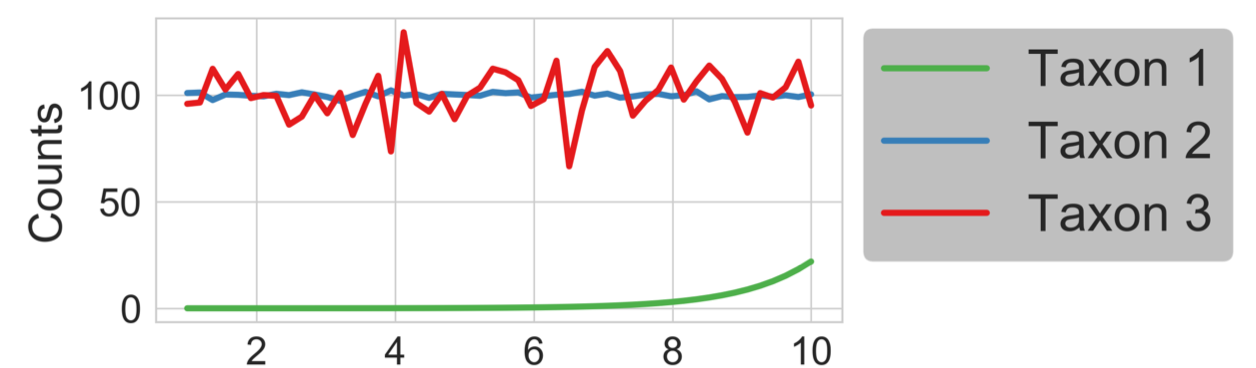In our distances below we have Euclidean, Bray-Curtis, Jaccard, and Aitchison distances (from left to right). We can see that the abundance based metrics Euclidean and Bray-Curtis are heavily influenced by the abundance of taxon 3 and seem to randomly fluctuate. In the presence/absence metric, Jaccard, we see that the distance saturates to one very quickly. However, in the Aitchison distance we see a linear curve representing taxon 1. The reason the distance is linear is because Aitchison distance relies on log transforms (the log of an exponential taxon 1 is linear).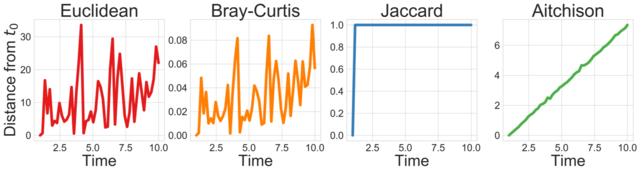From this toy example, it is clear that Aitchison distance better accounts for the proportions. However, we made the unrealistic assumption in our toy example that there were no zero counts. In real microbiome datasets there are a large number of zeros (i.e. sparsity). Sparsity complicates log ratio transformations because the log-ratio of zero is undefined. To solve this pseudo counts, that can skew results, are commonly used (see Naught all zeros in sequence count data are the same).

Robust Aitchison PCA solves this problem in two steps:

1. Compostional preprocessing using the centered log ratio transform on only the non-zero values of the data (no pseudo count)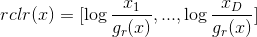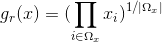2. Dimensionality reduction through Robust PCA on only the non-zero values of the data ( matrix completion).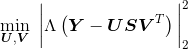To demonstrate this in action we will run an example dataset below, where the output can be viewed as a compositional biplot through emperor.

## Example¶

In this example we will use Robust Aitchison PCA via DEICODE on the “Moving Pictures” tutorial, if you have not yet completed the tutorial it can be found here. The dataset consists of human microbiome samples from two individuals at four body sites at five timepoints, the first of which immediately followed antibiotic usage (Caporaso et al. 2011). If you have completed this tutorial run the following command and skip the download section.

save as: table.qza

save as: taxonomy.qza

In :
!cd qiime2-moving-pictures-tutorial


Using table.qza, of the type raw count table (FeatureTable[Frequency]), we will generate our beta diversity ordination file. There are a few parameters to DEICODE that we may want to consider. The first is filtering cutoffs, these are p-min-feature-count, p-min-sample-count, and p-min-feature-frequency. Both p-min-feature-count and p-min-sample-count accept integer values and remove feature or samples, respectively, with sums below this cutoff. The feature cut-off is useful in the case that features with very low total counts among all samples represent contamination or chimeric sequences. The sample cut off is useful for the case that some sample received very few reads relative to other samples. The p-min-feature-frequency can be useful to remove features that only appear in a small portion of samples, which may be difficult to further asses using tools like Qurro.

Note: it is not recommended to bin your features by taxonomic assignment (i.e. by genus level). Note: it is not recommended to rarefy your data before using DEICODE.

The other two parameters are --p-rank and --p-iterations. These parameters should rarely have to change from the default. However, the minimum value of --p-rank can be 1 and the maximum recommended value is 10. Similarly, the minimum value of --p-iterations is 1 and is recommended to be below 500. The default value for p-min-feature-frequency is zero, meaning it will not be used.

The other main parameter of the DEICODE is the number of components to use (i.e. the rank). DEICODE relies on a low-rank assumption and therefore it is recommended to choose a value between 2 and 10. If you are unsure of what value to use for n-components the command auto-rpca will estimate it for you. In all other aspects the auto-rpca is the same as the rpca command.

Now that we understand the acceptable parameters, we are ready to run DEICODE.

In :
!qiime dev refresh-cache

QIIME is caching your current deployment for improved performance. This may take a few moments and should only happen once per deployment.

In :
!qiime deicode auto-rpca \
--i-table qiime2-moving-pictures-tutorial/table.qza \
--p-min-feature-count 10 \
--p-min-sample-count 500 \
--o-biplot qiime2-moving-pictures-tutorial/ordination.qza \
--o-distance-matrix qiime2-moving-pictures-tutorial/distance.qza

Saved PCoAResults % Properties('biplot') to: qiime2-moving-pictures-tutorial/ordination.qza
Saved DistanceMatrix to: qiime2-moving-pictures-tutorial/distance.qza


Now that we have our ordination file, with type (PCoAResults % Properties(['biplot'])), we are ready to visualize the results. This can be done using the Emperor biplot functionality. In this case we will include metadata for our features (optional) and our samples (required).

In :
!qiime emperor biplot \
--i-biplot qiime2-moving-pictures-tutorial/ordination.qza \
--o-visualization qiime2-moving-pictures-tutorial/biplot.qzv \
--p-number-of-features 8

Saved Visualization to: qiime2-moving-pictures-tutorial/biplot.qzv


Biplots are exploratory visualization tools that allow us to represent the features (i.e. taxonomy or OTUs) that strongly influence the principal component axis as arrows. The interpretation of the compositional biplot differs slightly from classical biplot interpretation (we can view the qzv file at view.qiime2. The important features with regard to sample clusters are not a single arrow but by the log ratio between features represented by arrows pointing in different directions. To effectively use Emperor we fist will color the samples by BodySite.Then by scrolling down the color selections, colors for arrows can be chosen based on taxonomy.Finally by toggling the colors on the legend, we can add custom coloring by the phylum.For a more detailed description see this discussion on the QIIME2 forum

From this visualization we noticed that BodySite seems to explain the clusters well. We can run PERMANOVA on the distances to get a statistical significance for this.

In :
!qiime diversity beta-group-significance \
--i-distance-matrix qiime2-moving-pictures-tutorial/distance.qza \
--p-method permanova \
--o-visualization qiime2-moving-pictures-tutorial/BodySite_significance.qzv

Saved Visualization to: qiime2-moving-pictures-tutorial/BodySite_significance.qzv


Indeed we can now see that the clusters we saw in the biplot were significant by viewing the BodySite_significance.qzv at view.qiime2.We can see from the biplot and PERMANOVA results that gut is very different from the skin samples. Next we can use qurro to explore log-ratios of the microbes highlighted by DEICODE. For more about why log-ratios are useful you may want to read "Establishing microbial composition measurement standards with reference frames".

In :
!qiime qurro loading-plot \
--i-ranks qiime2-moving-pictures-tutorial/ordination.qza \
--i-table qiime2-moving-pictures-tutorial/table.qza \

Saved Visualization to: qiime2-moving-pictures-tutorial/qurro_plot.qzv